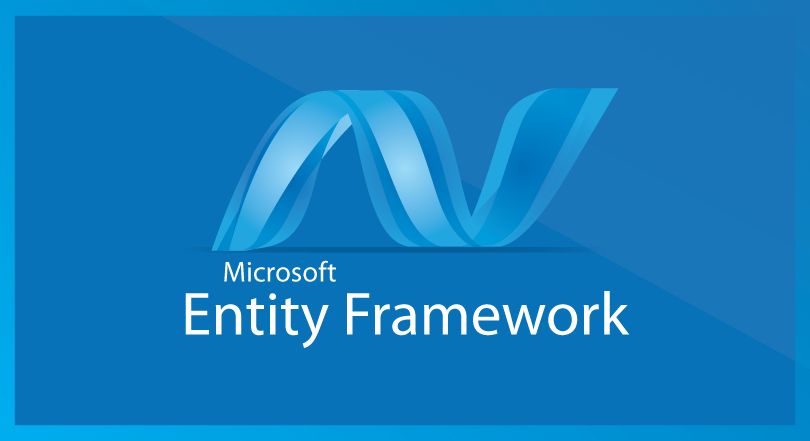### EF批量操作数据与缓存扩展框架

administrator / 2019/5/24 14:58 / 0 阅读 7460### 下载安装

`PM > Install - Package EntityFramework.Extended`
`框架安装后，你需要在您的类中是引用如下命名空间：`
`using EntityFramework.Extensions;`

### 批量更新与删除数据

```MyContext context = newMyContext();
context.Books.Where(b => b.Price >= 100).Delete();
context.SaveChanges();```

```MyContext context = newMyContext();
context.Books.Where(b => b.Price >= 100).Update(b => newBook { Price = 88 });
context.SaveChanges();```

### 批量查询

```MyContext context = newMyContext();

var books = context.Books.Where(b => b.Price >= 100).Future(); //生成第一个查询表达式
var accounts = context.Accounts.Where(a => a.Money <= 10).Future(); //生成第二个查询表达式

var bookList = books.ToList(); //在同一个数据库连接上查询上面两个表达式，只访问一次数据库```

```MyContext context = newMyContext();

var query = context.Books.Where(b => b.Price >= 100);
var query1 = query.Skip(pageIndex).Take(pageSize).Future();
var query2 = query.FutureCount();

var bookList = query1.ToList();
var bookTotalCount = query2.Value;```

### 缓存查询结果

EF扩展框架允许缓存查询结果，用法示例如下：

```MyContext context = newMyContext();

var books = context.Books.Where(b => b.Price >= 100).FromCache(); //不指定时间，使用默认的缓存时间
var books2= context.Books.Where(b => b.Price >= 100).FromCache(CachePolicy.WithDurationExpiration(TimeSpan.FromSeconds(300))); //将结果缓存300秒```
`当然，您也可以给缓存打上TAG标记，标记缓存的好处是，在以后的查询中可以设置指定的缓存过期或者获取指定TAG所缓存的数据，示例如下：`
```MyContext context = newMyContext();

var books = context.Books.Where(b => b.Price >= 100).FromCache(tags: new[] { "Books","100" });
CacheManager.Current.Expire("Books"); //将标记为Books的缓存立即过期```
`值得注意的是，EF扩展框架默认使用MemoryCache系统内存进行缓存，如果您想第三方分布式缓存框架，只需要移除系统内存缓存，注入自己的缓存提供者即可，如下用Memcache缓存结果。`
`Locator.Current.Register<ICacheProvider>(() => new MemcachedProvider());`

###### EF